﻿ 半球研磨均匀性三维仿真
«上一篇文章快速检索 高级检索

 哈尔滨工程大学学报2019, Vol. 40Issue (5): 1013-1017  DOI: 10.11990/jheu.2017111110

### 引用本文LU Jiangfeng, WANG Peng, LI Juan, et al. Simulation of hemispherical grinding uniformity based on model[J]. Journal of Harbin Engineering University, 2019, 40(5), 1013-1017. DOI: 10.11990/jheu.201711111.### 文章历史

1. 西北工业大学 航海学院, 陕西 西安 710072;
2. 西安航天精密机电研究所, 陕西 西安 710100

Simulation of hemispherical grinding uniformity based on model
LU Jiangfeng 1, WANG Peng 1, LI Juan 1, LU Jun 2, SHEN Kechun 1
1. School of Marine Science and Technology, Northwestern Polytechnical University, Xi'an 710072, China;
2. Xi'an Aerospace Precision Mechatronics Institute, Xi'an 710100, China
Abstract: An effective hemisphere grinding method-rotation machining with variable angles around a point is presented in this paper in order to improve lapping uniformity of spherical surface lapping path as well as to enhance the consistency and sphericity index of spherical surface processing. The established Preston function of hemisphere lapping indicates that the swing speed and rotational speed are the main factors affecting the material removal rate. Using the UG software, a 3D hemisphere simulation model was set up, and the movement pattern of hemisphere lapping was derived. On the basis of this movement pattern, the grinding process was simulated using the software Vericut, yielding a reasonable ratio of swing speed and rotational speed. Experimental results show clear improvement in the uniformity of hemisphere grinding. When the ratio of rolling speed to rotation speed is 2, the uniformity of hemispheric grinding is increased from 85% to 100%. The main findings of this paper have an important reference value and guidance function to hemisphere machining study.
Keywords: hemisphere    precision finishing    grinding    material removal rate    uniformity    motion curve    machining simulation

1 定心变角旋转加工原理及结构模型 1.1 半球加工原理

 $\mathrm{d} H / \mathrm{d} t=k p v$ (1)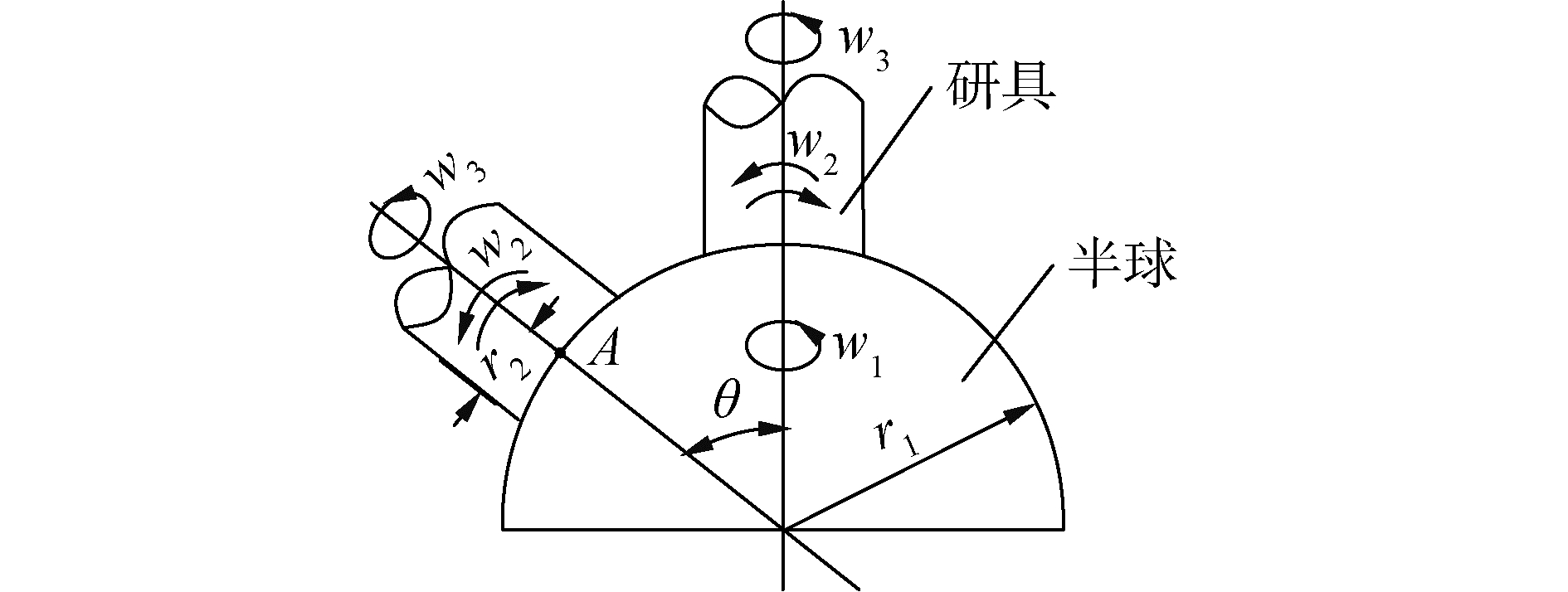Download: 图 1 研具与半球相对运动关系示意 Fig. 1 Diagram of relative motion relation between tool and hemisphere

 ${\mathit{\boldsymbol{v}}_1} = {\mathit{\boldsymbol{w}}_1}{\mathit{\boldsymbol{r}}_1}\sin \theta$ (2)

 $\boldsymbol{v}_{2} =\boldsymbol{w}_{2} \boldsymbol{r}_{1}$ (3)

 $\boldsymbol{v}_{3}=\boldsymbol{w}_{3} \boldsymbol{r}_{2}$ (4)

 $\mathit{\boldsymbol{v}} = \sqrt {{{\left( {{\mathit{\boldsymbol{v}}_1} - {\mathit{\boldsymbol{v}}_3}} \right)}^2} + \mathit{\boldsymbol{v}}_2^2}$ (5)

 ${\rm{d}}H/{\rm{d}}t = kp\mathit{\boldsymbol{v}} = kp\sqrt {{{\left( {{\mathit{\boldsymbol{v}}_1} - {\mathit{\boldsymbol{v}}_3}} \right)}^2} + \mathit{\boldsymbol{v}}_2^2}$ (6)

1.2 半球研磨机结构模型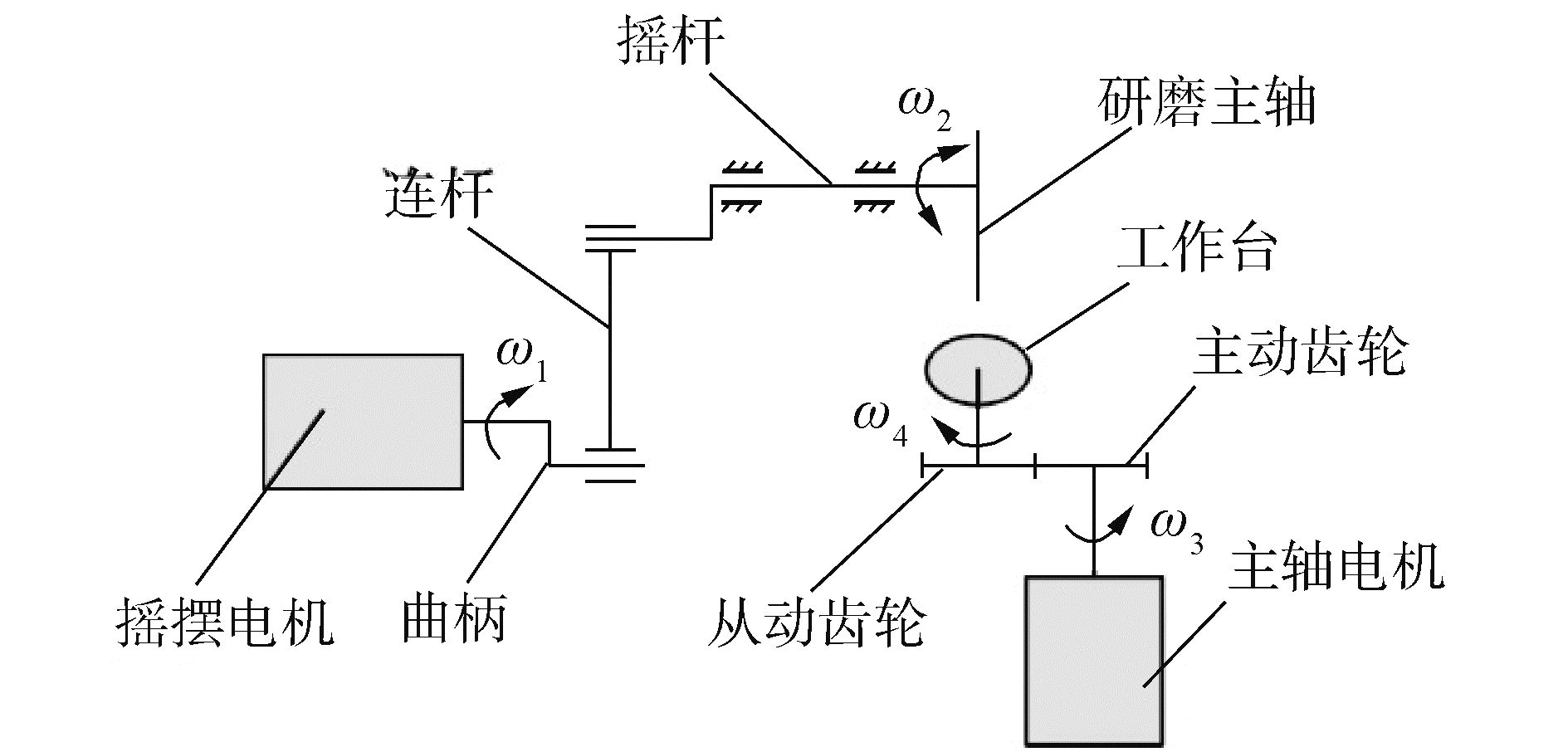Download: 图 2 研磨机运动原理 Fig. 2 Motion schematic diagram of grinding machine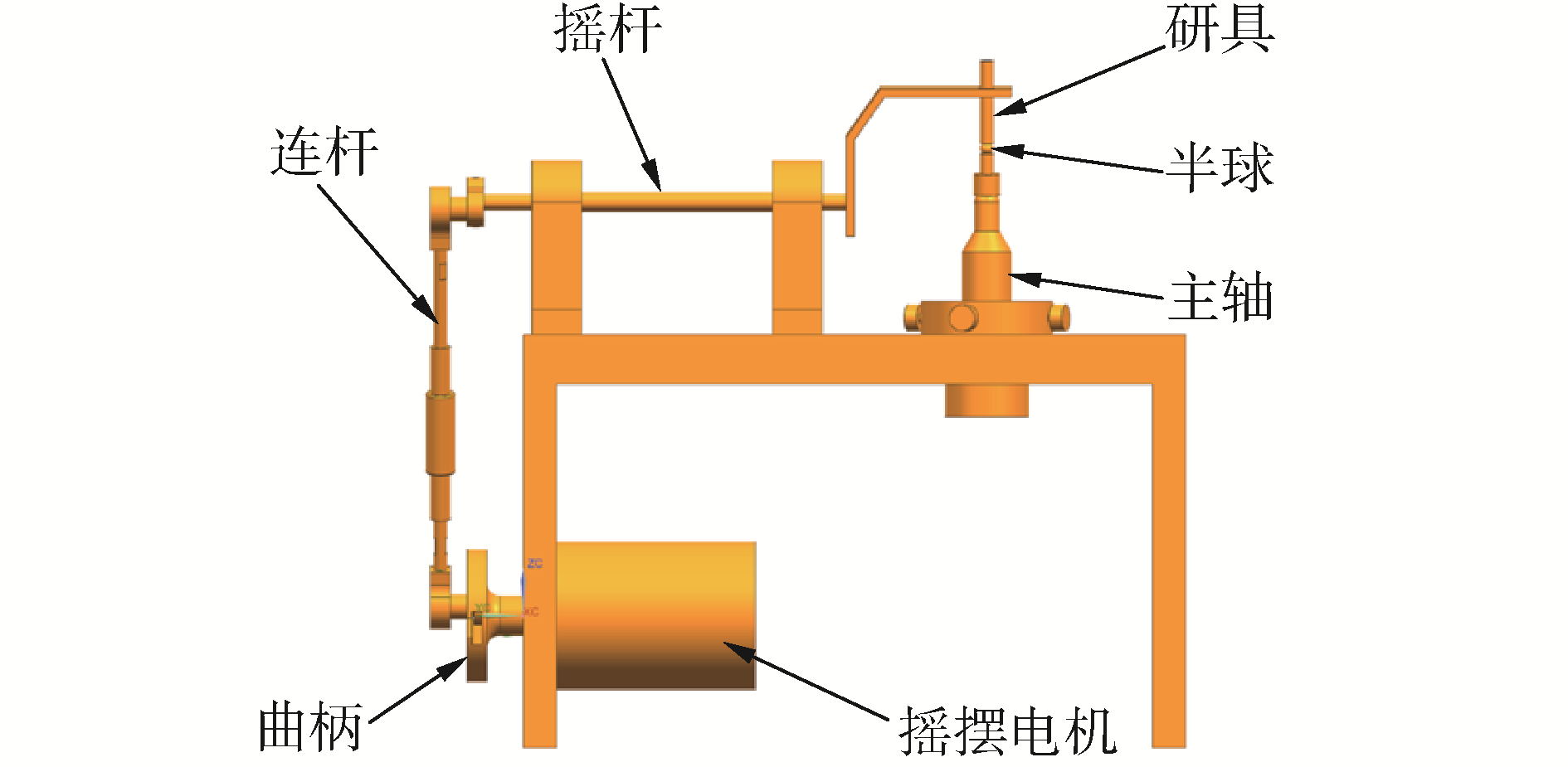Download: 图 3 研磨机三维示意 Fig. 3 3D diagram of grinding machine
2 研磨轨迹分析及仿真 2.1 研磨轨迹分析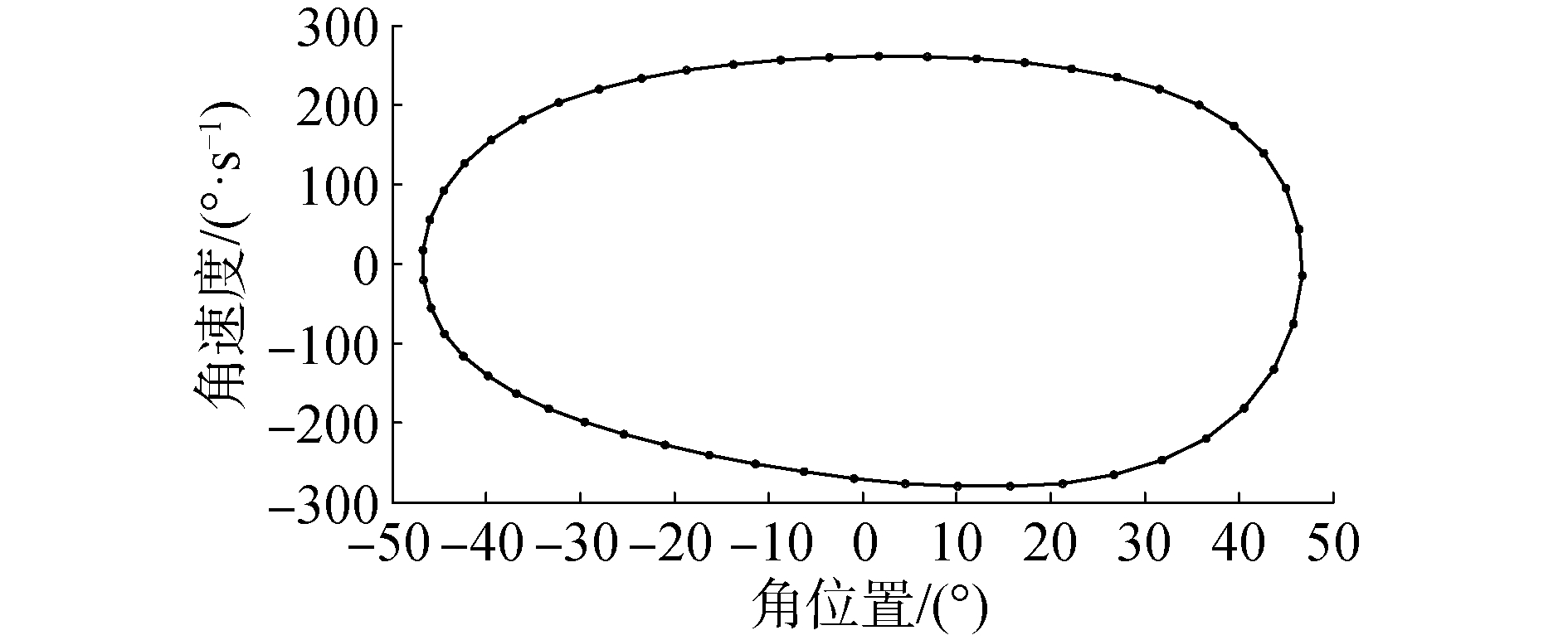Download: 图 4 研磨棒角速度—位置曲线 Fig. 4 Angular velocity and displacement curve of grinding tool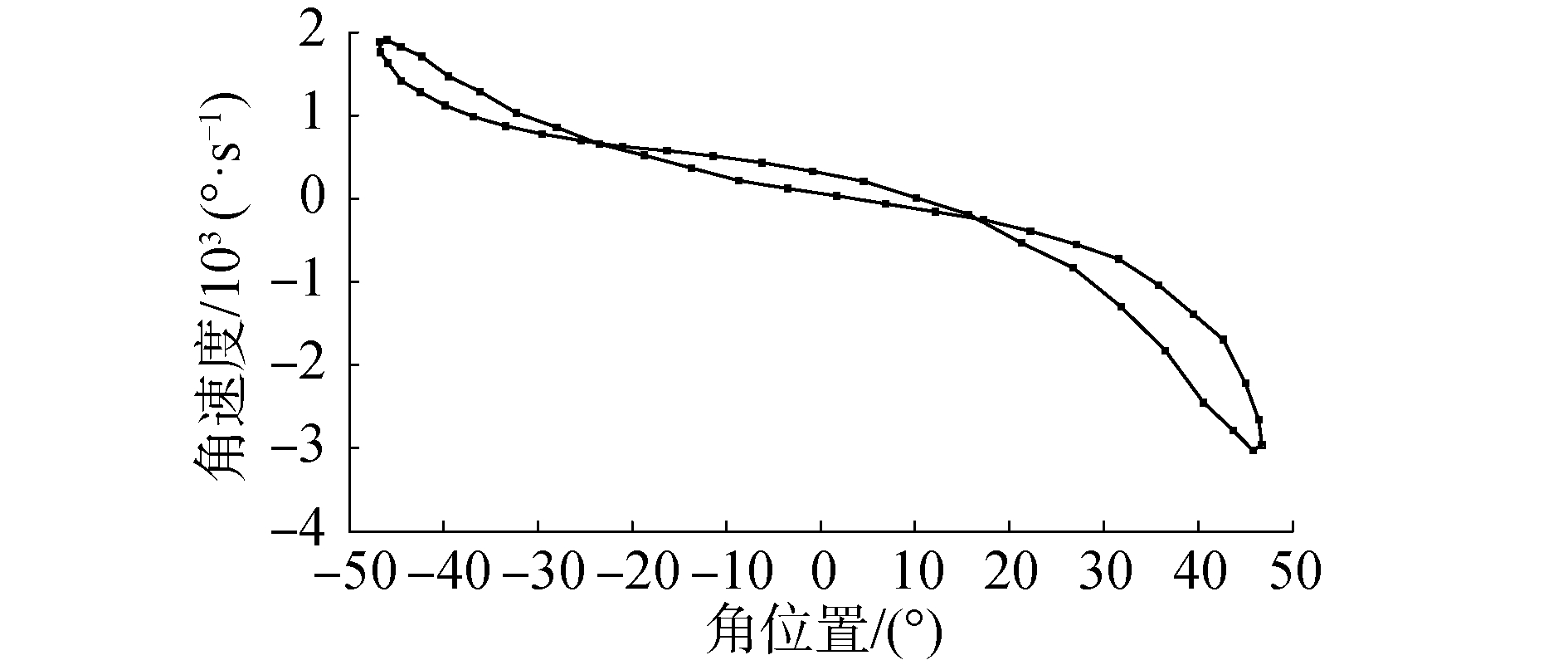Download: 图 5 研磨棒角加速度—位置曲线 Fig. 5 Angular acceleration and displacement curve of grinding tool

2.2 轨迹仿真Download: 图 6 研磨机加工仿真 Fig. 6 Machining simulation of grinding machine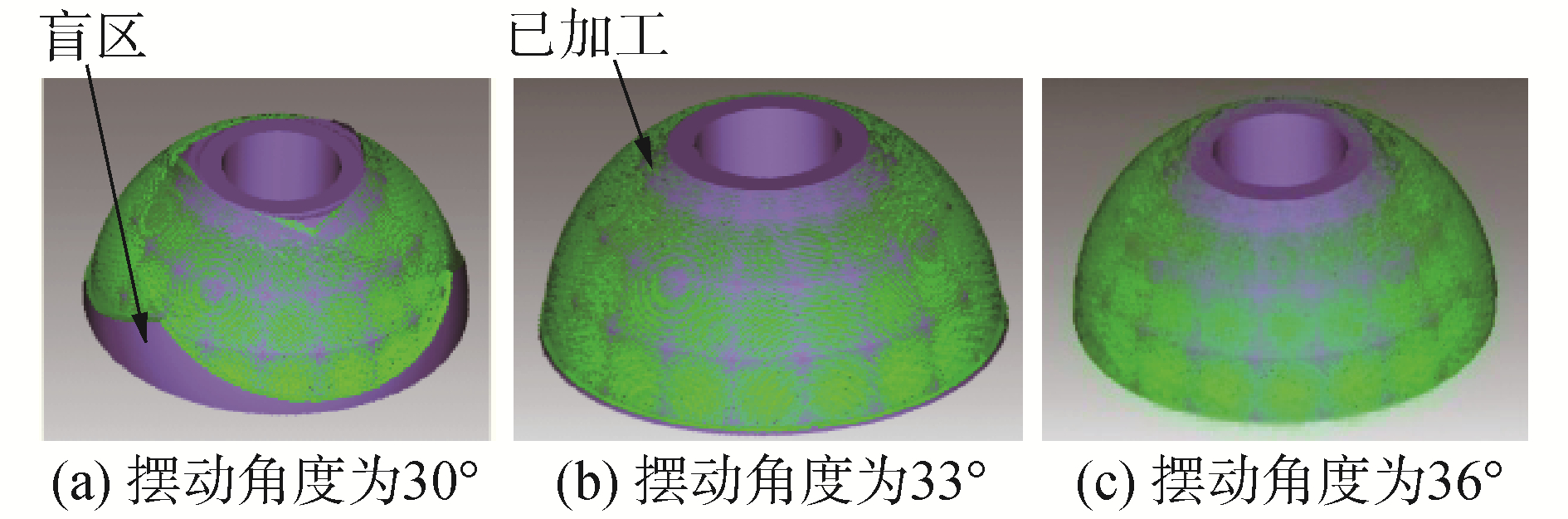Download: 图 7 摆动角度对球面加工的影响 Fig. 7 Influence of swing angle on machining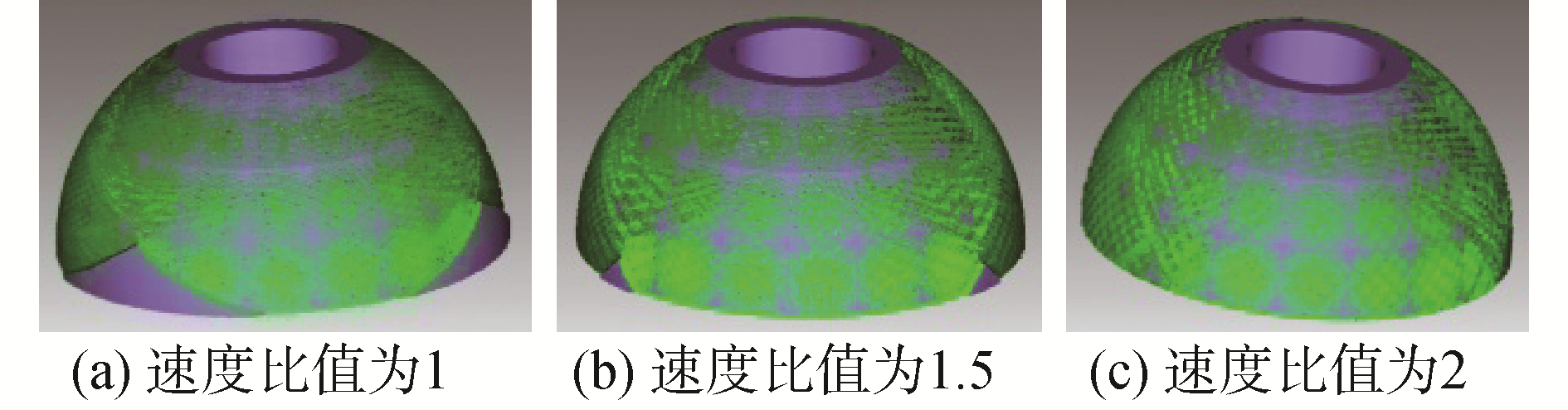Download: 图 8 速度比值对球面加工的影响 Fig. 8 Influence of velocity rate on machining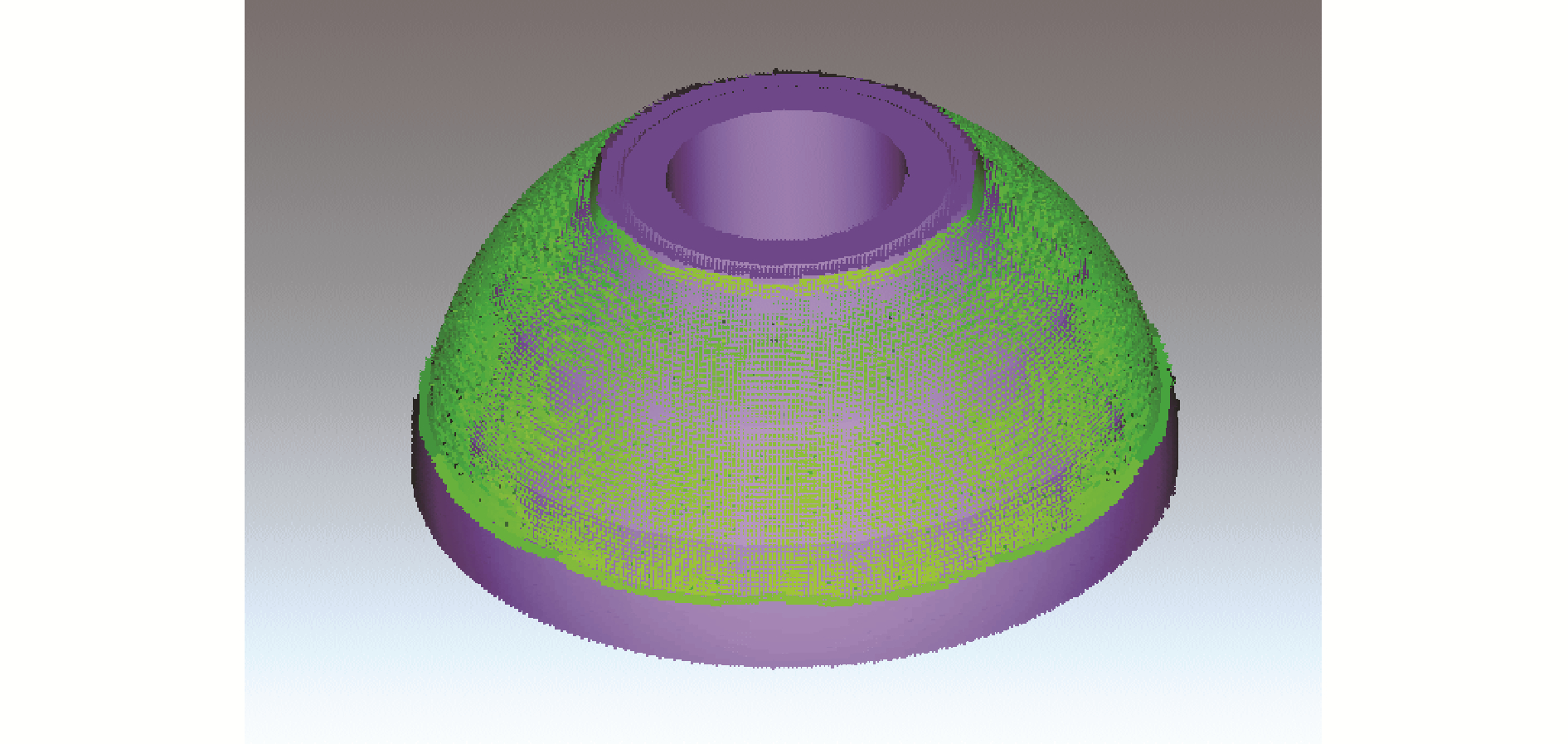Download: 图 9 研磨盲区效果 Fig. 9 Effect of grinding blind area

3 半球研磨试验验证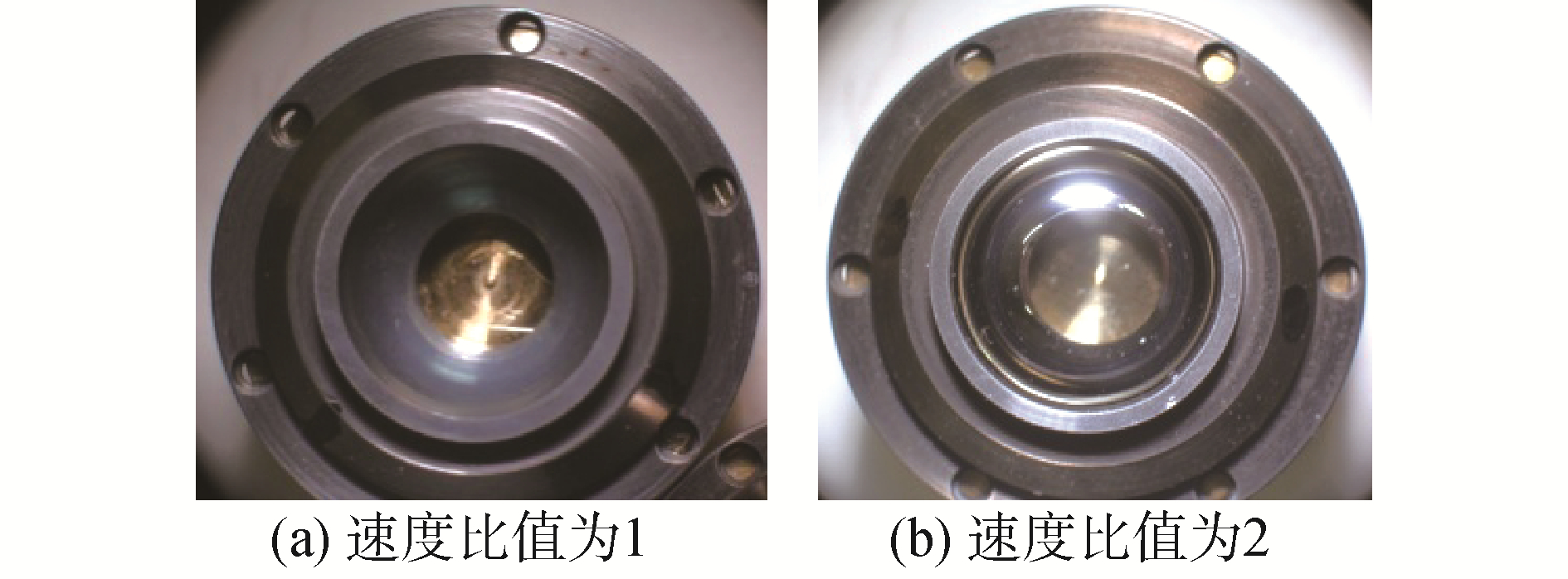Download: 图 10 不同速度比值下半球的研磨效果 Fig. 10 Hemisphere grinding effects of different velocity rate表 1 半球研磨数据对比表 Table 1 Hemisphere grinding data contrast

4 结论

1) 研磨棒摆动角度主要影响加工区域，角度小时存在研磨盲区，角度大时存在空走刀，36°为最佳的摆动角度。

2) 摆动速度和旋转速度比值影响加工区域的覆盖均匀性，当比值为2时，研磨棒覆盖球面的均匀性最好。

3) 半球研磨均匀性不仅包括加工区域的全覆盖，还包括研磨棒覆盖加工区域频率的一致性，必须同时满足前两条的最优，才能实现半球研磨均匀性的最优。

  王京锋, 刘景林, 闫亚超, 等. 半球型动压气体轴承陀螺电机设计及性能测试[J]. 哈尔滨工业大学学报, 2017, 49(9): 144-150. WANG Jingfeng, LIU Jinglin, YAN Yachao, et al. Design and performance testing of hemispheric aerodynamic bearing gyro motor[J]. Journal of Harbin Institute of Technology, 2017, 49(9): 144-150. (0)  YUAN J L, WANG Z W, LYU B H, et al. Simulation study on the developed eccentric V-grooves lapping mode for precise ball[J]. Key engineering materials, 2005, 304-305: 300-304. (0)  纪宏波, 刘成刚. 高精度球体研磨加工技术的现状和发展[J]. 机械研究与应用, 2016, 29(3): 205-207. JI Hongbo, LIU Chenggang. The situation and development of high-precision ball lapping technique[J]. Mechanical research & application, 2016, 29(3): 205-207. (0)  YAN Jiwang, TAMAKI J, SYOJI K, et al. Single-point diamond turning of CaF2 for nanometric surface[J]. International journal of advanced manufacturing technology, 2004, 24(9/10): 640-646. (0)  程相文, 王凌霄. 四轴球体研磨机研磨轨迹的研究[J]. 制造业自动化, 2013, 35(20): 98-100, 107. CHENG Xiangwen, WANG Lingxiao. Research on lapping trace of four cup lapping machine[J]. Manufacturing automation, 2013, 35(20): 98-100, 107. DOI:10.3969/j.issn.1009-0134.2013.20.028 (0)  张坤领, 韦建军. 基于Matlab的数控磨削球面粗糙度分析及仿真[J]. 组合机床与自动化加工技术, 2010(3): 16-21. DOI:10.3969/j.issn.1001-2265.2010.03.005 (0)  ZHANG Kunling, WEi Jianjun. Analysis and simulation of numerical control grinding spherical roughness based on Matlab[J]. Combined machine tool and automatic machining technology, 2010(3): 16-21. (0)  郁炜, 吕冰海, 姚蔚峰, 等. 基于ADAMS的球体双自转研磨方式下研磨盘转速优化研究[J]. 中国机械工程, 2013, 24(7): 866-872, 881. YU Wei, LYU Binghai, YAO Weifeng, et al. Speed optimization for lapping plates in RDP lapping mode based on ADAMS[J]. China mechanical engineering, 2013, 24(7): 866-872, 881. DOI:10.3969/j.issn.1004-132X.2013.07.004 (0)  YU W, LIU D, YUAN J L, et al. Processing of lapping track in dual rotated plates eccentric lapping mode[J]. Key engineering materials, 2011, 487: 248-252. DOI:10.4028/www.scientific.net/KEM.487 (0)  郑斌, 袁巨龙, 赵萍, 等. 变曲率沟槽精密球研磨加工优化实验研究[J]. 表面技术, 2017, 46(2): 214-219. ZHENG Bin, YUAN Julong, ZHAO Ping, et al. Experiment of optimized grinding of precision ball with variable-radius groove[J]. Surface technology, 2017, 46(2): 214-219. (0)  刘伟, 邓朝晖, 万林林, 等. 基于正交试验-遗传神经网络的陶瓷球面精密磨削参数优化[J]. 中国机械工程, 2014, 25(4): 451-455. LIU Wei, DENG Zhaohui, WAN Linlin, et al. Parameter optimization on precision grinding of ceramic sphere using orthogonal experiment and genetic neural network[J]. China mechanical engineering, 2014, 25(4): 451-455. DOI:10.3969/j.issn.1004-132X.2014.04.005 (0)  LIANG Fusheng, ZHAO Ji, JI Shijun, et al. Spherical approximation of free-form surface closed to a sphere in semi-finishing[J]. Key engineering materials, 2016(679): 199-206. (0)  郭伟刚, 袁巨龙, 项震, 等. 基于单转盘变曲率沟槽研磨方法的球体加工试验研究[J]. 表面技术, 2018, 47(7): 252-258. GUO Weigang, YUAN Julong, XIANG Zhen, et al. Spheroidal machining test based on variable turning curvature grinding method with single turntable[J]. Surface technology, 2018, 47(7): 252-258. (0)  PRESTON F W. The theory and design of plate glass polishing machines[J]. Journal of the society of glass technology, 1927(11): 214-256. (0)  周芬芬, 袁巨龙, 姚蔚峰, 等. 陶瓷球成球过程的建模与仿真[J]. 华中科技大学学报(自然科学版), 2016, 44(2): 128-132. ZHOU Fenfen, YUAN Julong, YAO Weifeng, et al. Modeling and simulation on sphere-shaping of ceramic balls[J]. Journal of Huazhong University of Science and Technology (natural science edition), 2016, 44(2): 128-132. (0)